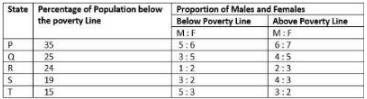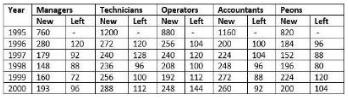Courses

# Test: Table Charts- 1

## 5 Questions MCQ Test General Aptitude for GATE 2020 | Test: Table Charts- 1

Description
This mock test of Test: Table Charts- 1 for LR helps you for every LR entrance exam. This contains 5 Multiple Choice Questions for LR Test: Table Charts- 1 (mcq) to study with solutions a complete question bank. The solved questions answers in this Test: Table Charts- 1 quiz give you a good mix of easy questions and tough questions. LR students definitely take this Test: Table Charts- 1 exercise for a better result in the exam. You can find other Test: Table Charts- 1 extra questions, long questions & short questions for LR on EduRev as well by searching above.
QUESTION: 1

### The following table gives the percentage distribution of population of five states, P, Q, R, S and T on the basis of poverty line and also on the basis of sex.Q. If the male population above poverty line for State R is 1.9 million, then the total population of State R is?

Solution:

Let the total population of state R be x million.
Then, population of state R above poverty line
= [(100 - 24)% of x] million
= (76 / 100 * x) million
And so, male populaion of state R above poverty line
= [2 / 5 x (76 / 100 * x)] million
But, it is given that male population of state R above poverty line = 1.9 million.
∴ 2 / 5 x (76 / 100 *x) = 1.9 ⇒ x = 5 x 100 x 1.9 / 76 x 2 = 6.25
∴ Total population of state R = 6.25 million.

QUESTION: 2

### The following table gives the percentage distribution of population of five states, P, Q, R, S and T on the basis of poverty line and also on the basis of sex.What will be the number of females above the poverty line in the State S if it is known that the population of State S is 7 million?

Solution:

Total population of state S = 7 million.
∴ Population above poverty line
= [(100 - 19)% of 7] million
= (81% of 7) million
= 5.67 million.
And so, the number of females above poverty line in state of S
= (3 / 7 x 5.67) million
= 2.43 million.

QUESTION: 3

### The following table gives the percentage distribution of population of five states, P, Q, R, S and T on the basis of poverty line and also on the basis of sex.What will be the male population above poverty line for State P if the female population below poverty line for State P is 2.1 million?

Solution:

Female population below poverty line for state P = 2.1 million.
Let the male population below poverty line for state P be x million.
Then, 5 : 6 = x : 21 ⇒ x = 2.1 x 5 / 6 = 1.75
∴ Population below poverty line for state P = (2.1 + 1.75) million = 3.85 million.
Let the population above poverty line for state P be y million
Since, 35% of the total population of state P is below poverty line.
Therefore, 65% of the total population of state P is above poverty line i.e., the ratio of population below poverty line to that above poverty line of state P is 35 : 65
∴ 35 : 65 = 3.85 : y ⇒ y = 65 x 3.85 / 35 = 7.15
∴ Population above poverty line for state P = 7.15 million and so, male population above poverty line for state P
= ( 6 / 13 x 7.15) million
= 3.3 million.

QUESTION: 4

The following table gives the percentage distribution of population of five states, P, Q, R, S and T on the basis of poverty line and also on the basis of sex.If the population of males below poverty line for State Q is 2.4 million and that for State T is 6 million, then the total populations of States Q and T are in the ratio?

Solution:

For state Q:
Male population below poverty line = 2.4 million.
Let the female population below poverty line be x million.
Then, 3 : 5 = 2.4 : x ⇒ x = 5 x 2.4 / 3 = 4.
∴ Total population below poverty line = (2.4 + 4) = 6.4 million.
If Nq = 6.4 million ⇒ Nq = (6.4 x 100 / 25) million = 25.6 million
For state T:
Male population below poverty line = 6 million.
Let the female population below poverty line be y million.
Then, 3 : 5 = 6 : y ⇒ y = 3 x 6 / 5 = 3.6.
∴ Total population below poverty line = (2.4 + 4) = 6.4 million.
If Nt = 6.4 million ⇒ Nt = (9.6 x 100 / 15) million = 64 million.
Thus, Required ratio = Nq / Nt = 25.6 / 64 = 0.4 = 2 / 5.

QUESTION: 5

The following table shows the number of new employees added to different categories of employees in a company and also the number of employees from these categories who left the company every year since the foundation of the Company in 1995.What is the difference between the total number of Technicians added to the Company and the total number of Accountants added to the Company during the years 1996 to 2000?

Solution:

Required difference

= (272 + 240 + 236 + 256 + 288) - (200 + 224 + 248 + 272 + 260)

= 88